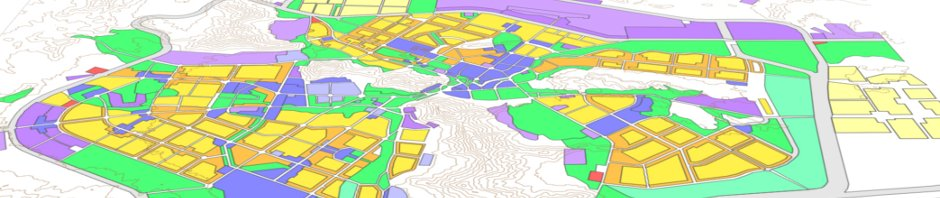# Import, derive new variables, export

This page explains some basic analysis and management of data compiled into a “master CSV” from Tables P5, DP02, DP03, and the DBF component of a shapefile for one county.
The method of creating this “master CSV” is explained on another page.

Start R-Studio. In this tutorial I am assuming that the workspace is completely empty of data-frames and other variables.
Set your Working Directory. You can do this “by hand” using the setwd() command, but it is generally easier to use the graphic menus:
Session > Set Working Directory > Choose Directory…
and then browse to the directory you want to use for automatic output of data. I chose:
~/soc393/Rstuff
Import {yourcounty}2010.csv  To use this tutorial, name your dataframe CO.
“CO” is a super-short name that stands for “county”, and if you use this name, you will be able to copy-and-paste many of the commands without even modifying them.

If you followed the instructions for compiling a “master-csv”, the newly imported data-frame “CO” should have 41 variables. The number of observations will equal the number of tracts in your county.

### Create two new variables & add them back into your dataframe: The logic of “building-up” R commands

If you managed to extract the area of each tract (in square meters) from the DBF file within the shapefile of your county, you can calculate the population density for each tract in people/hectare and people/acre. In this example, I am going to build up the R command by describing each step in the process.

First, we need to convert square meters into hectares and into acres. The basic equations are:
Area_SM / 10,000 = hectares
Area_SM / 4046.86 = acres

Second, the densities of each tract are calculated as the tract population divided by the area. In both cases, we will do this in one step:
TotTr / (Area_SM / 10000) = Dns_Ha
TotTr / (Area_SM/4046.86) = Dns_Ac

Third, I need to tell R to look for these variable inside dataframe “CO“, so I need to add the name of the dataframe as a sort of ‘address-prefix’ to the beginning of each variable:
CO\$TotTr / (CO\$Area_SM / 10000) = Dns_Ha
CO\$TotTr / (CO\$Area_SM / 4046.86) = Dns_Ac
These equations would work in R. They would create the freestanding named variables Dns_Ac and Dns_Ac. But I don’t want to create freestanding variables. I want to write them back into the dataframe so that I can easily extract them from R with the write.csv(CO, “backup.csv”) function. That way I will have a backup, but also I can use the data in other software. So:

Fourth, I want to pipe the calculations back into new, named columns in my dataframe. That means using the following syntax: function -> CO[“NewVariableName”]
Here, then, are the full-blown commands to do this in R:

```CO\$TotTr / (CO\$Area_SM / 10000) -> CO["Dns_Ha"]
CO\$TotTr / (CO\$Area_SM / 4046.86) -> CO["Dns_Ac"]```

Now, you can copy these commands (formatted in Courier font) and paste them into your own R-Studio session and run them. As a standard practice I will show commands in Courier, with a light gray background.

### Create proportions and percentages of each population within each tract

Using this same syntax, I am going to generate a series of proportions of groups within each tract:

```CO["prpWhtTr"] <- CO\$WhtTr / CO\$TotTr
CO["prpBlkTr"] <- CO\$BlkTr / CO\$TotTr
CO["prpNtvTr"] <- CO\$NtvTr / CO\$TotTr
CO["prpAsnTr"] <- CO\$AsnTr / CO\$TotTr
CO["prpApiTr"] <- CO\$ApiTr / CO\$TotTr
CO["prpOthTr"] <- CO\$OthTr / CO\$TotTr
CO["prpMltTr"] <- CO\$MltTr / CO\$TotTr
CO["prpLatTr"] <- CO\$LatTr / CO\$TotTr```

Note that I am using an arrow (<-) indicator, and it points toward the new variable that is being created. You could use the equals sign (=) and let R figure out which side is a function it needs to perform on existing variables, and which side is a new variable that needs to be created and populated with the output from the function.

Second, I will multiply these raw proportion numbers by 100 to create percentage data. Sometimes these can be more useful in later analysis, because they are more intuitive to interpret.

```CO["pctWhtTr"] <- CO\$prpWhtTr * 100
CO["pctBlkTr"] <- CO\$prpBlkTr * 100
CO["pctNtvTr"] <- CO\$prpNtvTr * 100
CO["pctAsnTr"] <- CO\$prpAsnTr * 100
CO["pctApiTr"] <- CO\$prpApiTr * 100
CO["pctOthTr"] <- CO\$prpOthTr * 100
CO["pctMltTr"] <- CO\$prpMltTr * 100
CO["pctLatTr"] <- CO\$prpLatTr * 100```

Remember, you can copy each of the commands straight off this webpage, paste them onto the command-line of R (lower left in R-Studio), adjust for the shorthand name you have given your county, and then run the command. However, you might want to paste all of these into a script window in the upper-left Source Pane of R-Studio. Then, when you run successful commands, you are also building a record of each successful function. You can also run multiple commands by highlighting them in the Scripts window and clicking Run.

### Export the dataframe using the write.csv() command

Now that you have derived a new set of variables in R, it is time to backup this dataframe. We will use the write.csv() command to export the data as a CSV file, with a name that we choose. My recommendation is that you choose a filename that includes the date, so that you will know exactly when this export was done. On this day, I would use “CO_141221.csv” as my exported-file name. In generic terms, this would be “CO_{YYMMDD}.csv”, and you replace everything within (and including) the curly-braces. Here is the exact command:

`write.csv(CO, "CO_141221.csv")`

The next page is devoted to error-correction in R, along with more info about backing up and exporting data and commands from R.